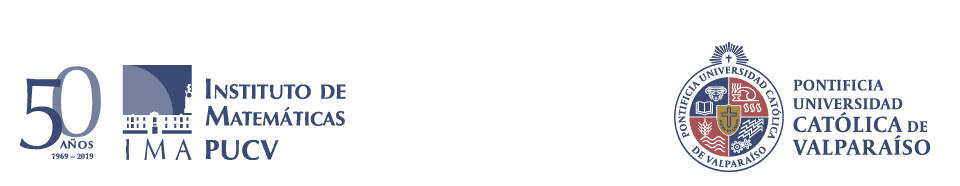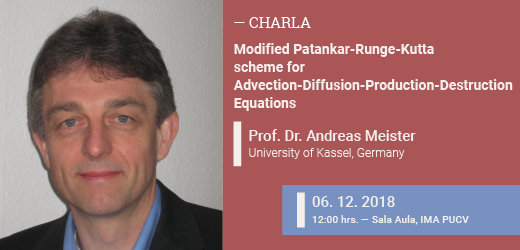﻿ Modified Patankar-Runge-Kutta scheme for Advection-Diffusion-Production-Destruction Equations | Instituto de MatemáticasModified Patankar-Runge-Kutta scheme for Advection-Diffusion-Production-Destruction EquationsModified Patankar-Runge-Kutta scheme for Advection-Diffusion-Production-Destruction Equations

Modified Patankar-Runge-Kutta (MPRK) schemes are numerical methods for the solution of positive
and conservative production-destruction systems.
They adapt explicit Runge-Kutta schemes in a way to ensure positivity and conservation irrespective of the time step size.

We introduce a general definition of MPRK schemes and present a thorough investigation of necessary as well as sufficient
conditions to derive first, second and third order accurate MPRK schemes. The
theoretical results will be confirmed by numerical experiments  in which MPRK schemes are applied to solve non-stiff
and stiff systems of ordinary differential equations.

Furthermore, we present an investigation of MPRK schemes in the context of convection-diffusion-reaction equations with source
terms of production-destruction type.

Compartir esta información en:

Compartir